One-Parametric Deformations of Solids

1. Introduction

Solid cell T is a non-empty subset of the projectively extended Euclidean space over the set of real numbers ¥E3 that is the image of a simply connected region W = [0, 1] 3 Ì R3 under a local homeomorphism, mapping analytically represented by the point function

s(ui) = (x(ui), y(ui), z(ui), h(ui)),  for  i = 1, 2, 3, ui Î W.

In the solid cell regular point P = s(a, b, c), (a, b, c) Î W, the tangent trihedron is uniquely determined and describing solid intrinsic geometric properties, see in , . Vertex of the tangent trihedron is in the regular point P, the common point of the tangent trihedron edges in semi-lines determined by the regular point  P and three direction vectors

si(u1, u2, u3)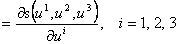,

which are linearly independent tangent vectors to the solid cell iso-parametric curve segments located in the point P. Faces of the tangent trihedron are parts of three tangent planes to the solid iso-parametric surface patches sharing the common regular point P. Twist vectors

sij(u1, u2, u3)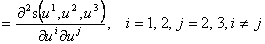to these solid cell iso-parametric surface patches are located in the tangent trihedron.

Interior distribution of the solid cell is indicated by the solid cell density vector

s123(u1, u2, u3)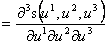.

The first differential form of a solid cell in the regular point P=s(a,b,c), (a,b,c)ÎW, can be determined (see in )

(ds)2 = (sidui) 2 = sisj duiduj =,

and the discriminant of the solid cell first differential form can be represented as

D = EGG* + 2FF*F** - GF*2 - F2G* - EF**2  = A + 2C - B,

A = EGG*, C = FF*F**, B = GF*2 + F2G* + EF**2.

The mixed triple scalar product

s = [s1 s 2 s 3] = (s1´s2).s3 = [s2 s3 s1] = (s2´s3).s1= [s3 s1 s2] = (s3´s1).s2

of the tangent vectors s1, s2,  s3 to the solid cell iso-parametric curve segments satisfies the equation (as proved in )

s2 = D.

Approximation Bèzier tri-cubic solid cell is determined by the ordered basic grid of 64 real points in the space. Map of the basic figure is the matrix M4x4x4 with elements in the homogeneous co-ordinates of points in the basic grid

M4x4x4 = (ijkP),  i, j, k = 0, 1, 2, 3.

Tri-cubic approximation is determined by Bernstein polynomials for a = 1, 2, 3

Be0(ua)=(1 - ua)3       Be1(ua)=3ua(1 - ua)2       Be2(ua)=3ua2(1 - ua)      Be3(ua)=ua3

and interpolation matrices are in the form

aI(ua) = (Be0(ua), Be1(ua), Be2(ua), Be3(ua)),  a=1, 2, 3.

Modelled Bèzier cell has vertices in the vertices of the basic grid, while no other point of the basic figure is located in the cell. Point function of the cell is in the form

s(u1, u2, u3) = 3I(u3) . (1I(u1) . M4x4x4 . 2I(u2)T)= ijkPBei(u1)Bej(u2)Bek(u3), i, j, k = 0, 1, 2, 3.

Density vector s123(a, b, c) in the cell arbitrary point P(a, b, c), for a, b, c Î [0, 1] is determined by points of the basic grid and can be calculated from the density function

s123(u1, u2, u3) = 3I´(u3).(1I´(u1).M4x4x4.2I´(u2) T) = ijkPBei´(u1)Bej´(u2)Bek´(u3), i, j, k = 0, 1, 2, 3

aI´(ua)=(Be0´(ua), Be1´(ua), Be2´(ua), Be3´(ua)), a=1, 2, 3

Be0´(ua) = -3(1 - ua)2,  Be1´(ua) = 3(1-ua )(1 - 3ua),  Be2´(ua) = 3ua (2 - 3ua),  Be3´(ua) = 6ua2

which is the polynomial function of degree two in three variables, where ijkP are points of the basic grid, elements of the map M4x4x4.

Density vectors in the vertices of the Bèzier cell (with parametric coordinates equal to 0 or 1) do not change their position while considering interior deformations, and therefore only 8 interior points (one for each vertex) of the basic grid determine entire interior solid cell geometry and density. These points form interior density core, determined as interior density hypercube, which influences all interior density. Density core can be deformed arbitrarily (as discussed in ) to create a desirable distribution in the net of iso-parametric curve segments and surface patches, and to determine the interior density of the modelled solid cell and its in-homogeneity in this way. Minor deformations of the homogeneous distribution of iso-parametric curve segments in the cell can be reached only, while moving all vertices of the interior density hypercube within the convex envelope of the Bèzier solid cell basic grid of 64 real points in the matrix M4x4x4 . Extreme changes can be adopted by moving these points outside the Bèzier solid cell basic grid envelope. A special class of deformations is discussed, called one-parametric deformations, based on the translation of control points in the direction of one from parameters ui Î [0, 1], for  i = 1, 2, 3.

Let the solid cell point function can be rewritten in the way

s(u1, u2, u3) =  3I(u3) . (1I(u1) . M4x4x4 . 2I(u2)T), (u1, u2, u3) Î W.

The three dimensional matrix M4x4x4 contains control points of the solid basic grid, including 8 interior points forming the interior density hypercube, but these points are attached a weight, so cold density parameter influencing the solid interior density. This means, points ijkP, i, j, k = 2, 3 are substituted by points ijkP, = ijkh. ijkP, ijkh being the nonzero weights.

We can write therefore

s(u1, u2, u3) =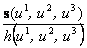, (u1, u2, u3) Î W,

where the function h(u1, u2, u3) can be expressed as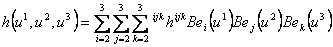, (u1, u2, u3) Î W.

Inserting extremal values of the weights to the vertices of the solid interior density hypercube, a special interior density can be reached.

In the figure 1 below, there are illustrations of the inhomogeneous interior distribution of the iso-parametric net of surfaces in the solid cell, which is an in-homogeneous cube, a rational Bèzier solid cell. There had been chosen a special position of the vertices of the interior density hypercube. Its four "bottom" vertices, 4 control points in the second level of the solid basic grid, were located into one point, while in the same way, the remaining four "top" vertices of the hypercube, it means 4 control points in the third level of the solid basic grid were also located in one point only. Also the corresponding weights were chosen equal, only their different and changing signs had been tested with respect to the possible combinations and their influence on the interior density distribution of the iso-parametric net of surfaces.

If  ijkh.> 0 for i, j = 2, 3 and k = 2, and  ijkh.> 0 for i, j = 2, 3 and k = 3, we can speak about concave behaviour of iso-parametric surfaces in the solid interior (situation in the upper left corner at the Fig. 1), while for the negative values of the related weights we can see their convex behaviour (situation in the upper right corner in the Fig. 1).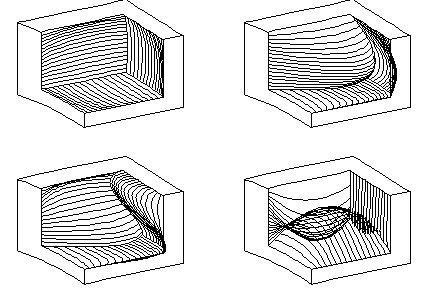Fig. 1

In the case of  ijkh.> 0 for i, j = 2, 3 and k = 2, and  ijkh.< 0 for i, j = 2, 3 and k = 3, we can speak about iso-parametric surfaces forming "knots" - which means self-intersections of these surfaces (situation in the bottom left corner at the Fig. 1), while in the case of  ijkh.< 0 for i, j = 2, 3 and k = 2 and  ijkh.> 0 for i, j = 2, 3 and k = 3, we can speak about bubbles that might appear in the distribution of iso-parametric surfaces (situation in the bottom right corner at the Fig. 1).

More details on the possible modelling of the solid interior density with respect to the given form of the iso-parametric surfaces and their common position will be obtained in the future further study of the relevant problems with relations to the solid first differential form and its discriminant.